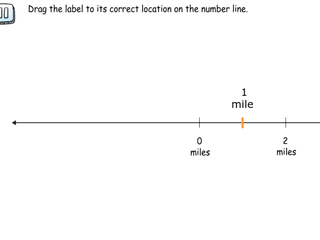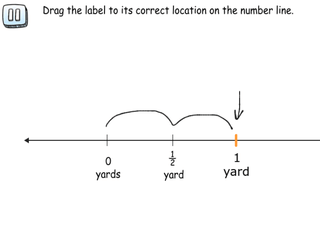# Interactive Problem Bank

•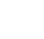Fraction Circles
•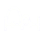Number Lines
•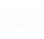Fraction Bars
•Sets
•Area Models
•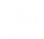Base Ten Blocks

## Fractions on a Number Line

Students understand a fraction as a number on the number line, label a number line using fraction notation, and place fractions on a number line in a variety of real-world contexts. Fractions are less than 1, between 1 and 2, and whole numbers written in fraction form.

### Introducing 1/b on the Number Line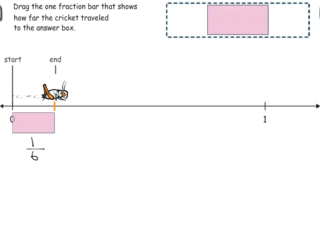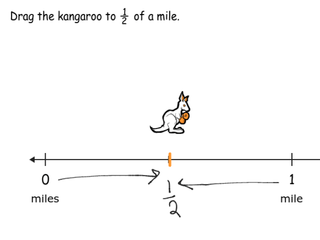### Introducing Partitioning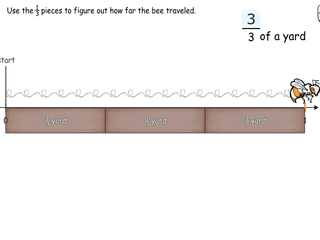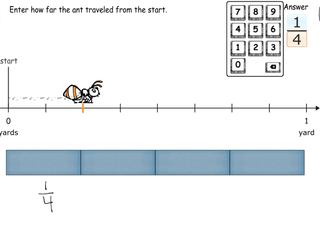### Partitioning the Number Line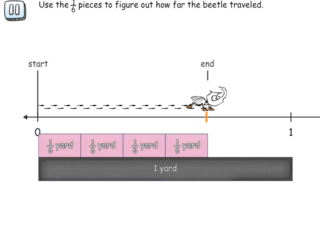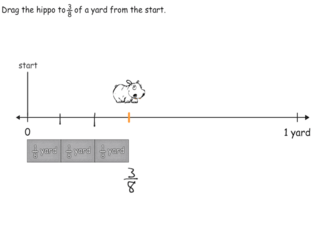### Labeling Number Lines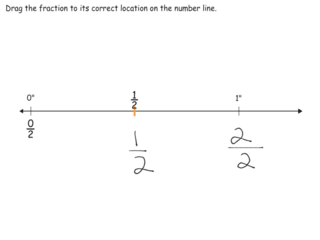### Length on a Number Line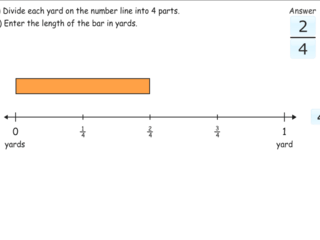### Placing Fractions on a Number Line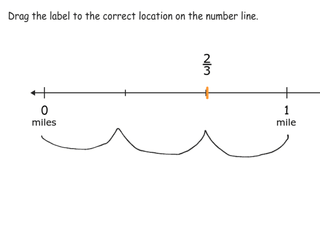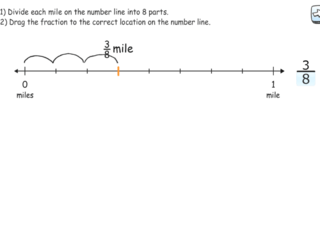### Placing Fractions Between 1 and 2 on a Number Line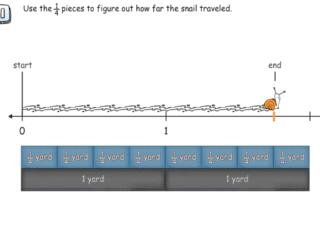### One as a Fraction on the Number Line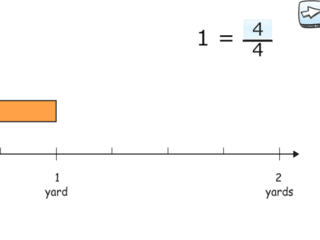### Locating 0 and 1 on the Number Line